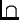Next: Computer Graphics and Image Up: Easter Term 2002: Part Previous: Easter Term 2002: Part   Contents

## Complexity Theory

Lecturer: Dr A. Dawar (ad260@cl.cam.ac.uk)

No. of lectures: 12

Prerequisite courses: Data Structures and Algorithms, Computation Theory

Aims

The aim of the course is to introduce the theory of computational complexity. The course will explain measures of the complexity of problems and of algorithms, based on time and space used on abstract models. Important complexity classes will be defined, and the notion of completeness established through a thorough study of NP-completeness. Applications to cryptographic protocols will be considered.

Lectures

• Algorithms and problems. Complexity of algorithms and of problems. Lower and upper bounds. Examples: sorting and travelling salesman.

• Time and space. Models of computation and measures of complexity. Time and space complexity on a Turing machine. Non-deterministic machines. Decidability and complexity.

• Complexity classes. Complexity classes. P, NP and PSPACE, LOGSPACE and their inter-relationships.

• Hierarchy. The time and space hierarchy theorems and complete problems.

• Reachability. Non-deterministic space complexity. The reachability method. Savitch's theorem.

• Boolean logic. The complexity of propositional logic. The circuit value problem and Boolean satisfiability.

• NP-completeness. Reductions and completeness. NP-completeness of satisfiability.

• More NP-complete problems. Graph-theoretic problems. Hamiltonian cycle and clique.

• More NP-complete problems. Sets, numbers and scheduling. Matching, set covering and bin packing.

• coNP. Validity of Boolean formulas and its completeness. NPcoNP. Primality. Pratt's algorithm.

• Cryptographic complexity. One-way functions. The class UP.

• Protocols. Interactive proofs. Zero knowledge.

Objectives

At the end of the course students should

• be able to analyse practical problems and classify them according to their complexity

• be familiar with the phenomenon of NP-completeness, and be able to identify problems that are NP-complete

• be aware of a variety of complexity classes and their interrelationships

• understand the role of complexity analysis in cryptographic protocols

Recommended book

Papadimitriou, Ch.H. (1994). Computational Complexity. Addison-Wesley.Next: Computer Graphics and Image Up: Easter Term 2002: Part Previous: Easter Term 2002: Part   Contents
Christine Northeast
Tue Sep 4 09:34:31 BST 2001